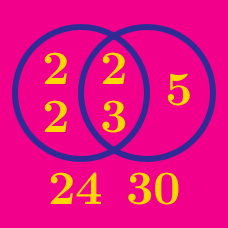Number Theory

# GCD and LCM - Problem solving

If the lowest common multiple of $$4,6,8,9,$$ and $$x$$ is $$504,$$ what is the third smallest possible integer $$x?$$

Note: Third smallest means the third value of $$x$$ if the possible values were listed from least to greatest.

What is the largest 3-digit number that is a multiple of 12 and a multiple of 21, but not a multiple of 22?

If the greatest common divisor of $$266,308,392,$$ and $$x$$ is $$7,$$ what is the sum of the smallest and largest possible 2-digit positive integers $$x?$$

$$A$$ and $$B$$ are positive integers such that $\begin{array} &\gcd(A,B)= 9, &\mbox{lcm}(A,B) = 180, &A<B. \end{array}$ How many such $$A$$'s are there?

What is the lowest common multiple of the numbers 8, 9, 10, and 12?

×

Problem Loading...

Note Loading...

Set Loading...